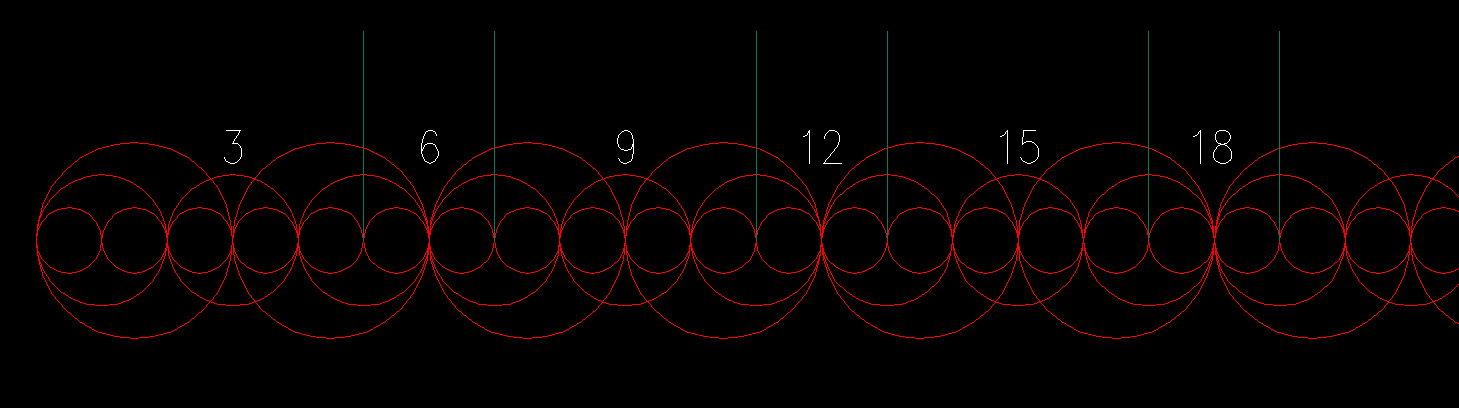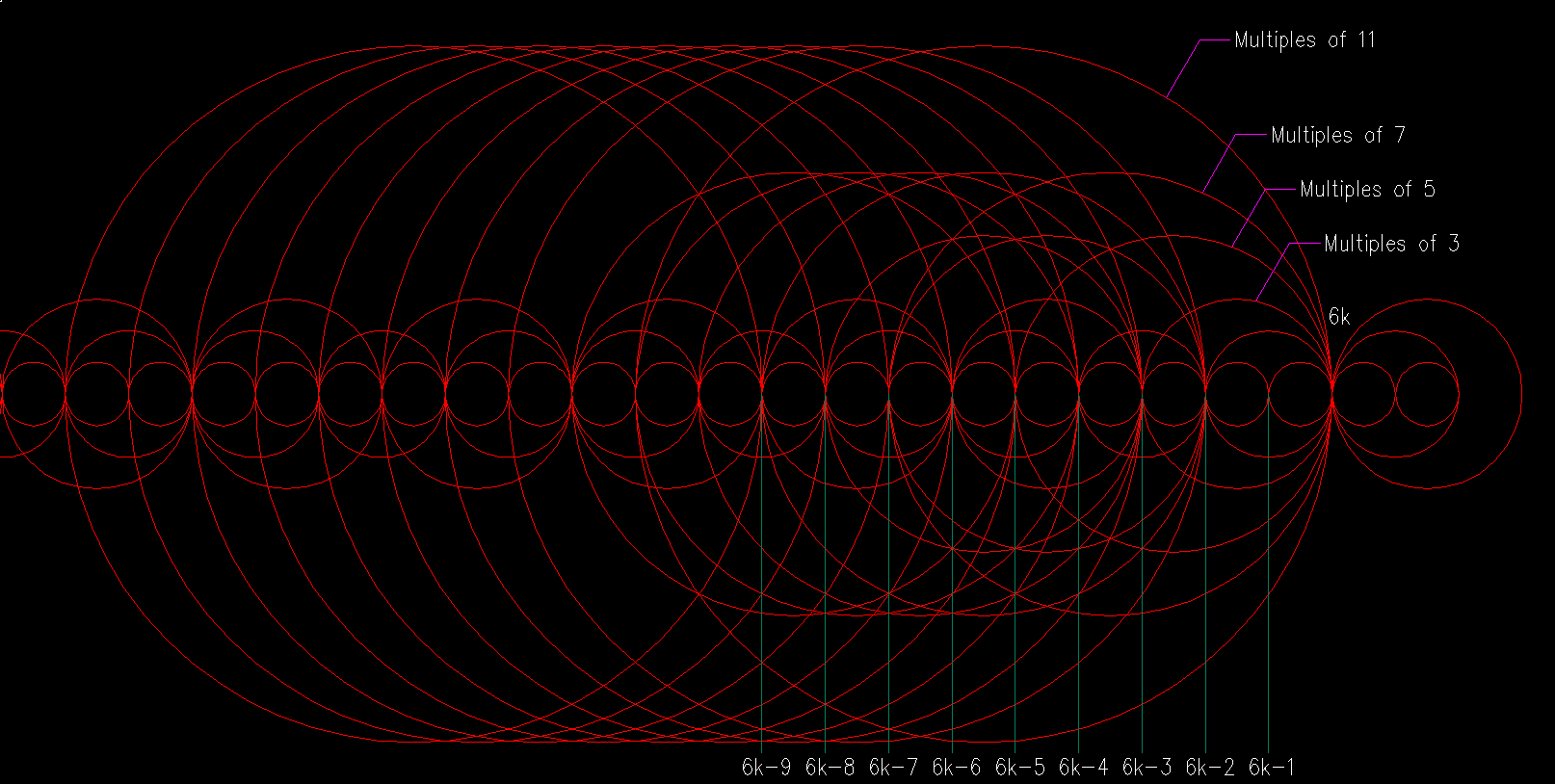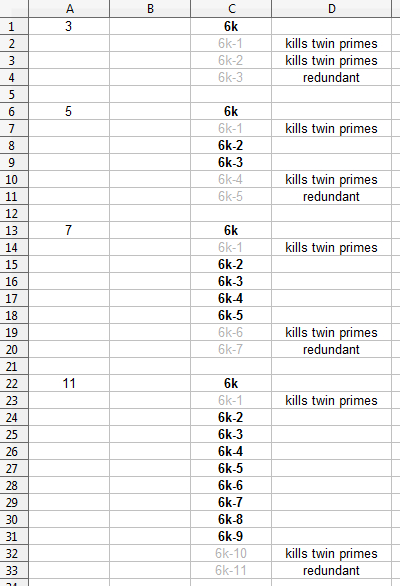# A Clockwork Prime

I'm going walk you through the mental process I'm still undertaking, and explain how I see prime numbers occur, especially twin primes.

Figure 1 shows multiples of 1, 2 and 3:This image shows that, as a general rule, prime numbers hover around multiples of the number 6. You may argue that a prime number hovers around 10 (11 is prime) for example, however, 6 is the only number that can  have prime numbers to both of its sides (11 hovers around 12 too, which is a multiple of 6). So, it is obvious that all prime numbers will always be of the form 6k + 1 or 6k - 1, where k is the iteration that will give us multiples of 6.

Twin primes specifically are always of the form 6k +/- 1. For example, the twin primes 29 and 31 are of the form 6x5 -1 = 29, and 6x5+1=31.

With this reasoning, I asked myself how the multiples of any number fit into multiples of 6 so as to ALLOW for twin primes to occur. To visualize this I drew Figure 2:The image shows a random multiple of 6 at "6k". In order to have twin primes we cannot have any circle intersecting adjacently to 6k (obviously). Let's see how this happens:

Please note that the reason I'm only using prime numbers for this is because we only need prime numbers. For example, the 9 is not needed because it is a multiple of 3 (3 is prime and can only fall on 6k). Same with 15, 21, 27 and so on (which are also multiples of 3). Same applies with multiples of 5, 7, 11, etc. They are not needed.

-Multiples of 3 can only fall on 6k.

-Multiples of 5 can fall on 6k, 6k-2, 6k-3. Notice if we put a 5 at 6k-4, then the circle will fall on 6k+1, which would kill the twin primes. Any other possibility for the number 5 is redundant and not needed, for example, having the 5 circle fall on 6k-5 is the same as having it fall on 6k. Let's keep doing this for 7 & 11.

-Multiples of 7 can fall on 6k, 6k-2, 6k-3, 6k-4, 6k-5. Notice if we put a 7 at 6k-6, then the circle will fall on 6k+1, which also kills the twin primes. Again, there is no need for more possibilities because they would be redundant.

-Similarly Multiples of 11 can fall on 6k, 6k-2, 6k-3, 6k-4, 6k-5, 6k-6, 6k-7, 6k-8, 6k-9.

Let's put this in a chart of sorts. On the right you will see the possible locations of the number around multiples of 6:The values in bold are the positions of that number that allow for twin primes.

As you can see, any prime number "p" has "p - 2" possible locations that allow for twin primes. Now, what I did next was arrange the chart for each number in a circular form, resembling the hours in a clock. Figure 3 shows this arrangement for the numbers 5, 7 and 11 (remember to scroll to the right):The blue radial lines show the locations that do not allow for twin primes.  As you can see, each number has its own clock, in which the hand rotates counter-clockwise six places for each multiple of the number 6. For example, at the first multiple of 6 (6 x 1=6) the hand of the 5 clock is located at 6k-1 or at 6k+4 (6 - 1 = 5  or  6 + 4 = 10). At the second multiple of 6 (12), the hand of the 5 clock moves counter-clockwise 6 places and lands on 6k-2 or at 6k+3 (12 - 2 = 10 , 12 + 3 = 15).

As long as we know the initial locations of the hands corresponding to the 5 clock, 7 clock, 11 clock , etc. we know exactly where that number will fall for each multiple of 6. The hands of all the clocks always move six places counter-clockwise for each multiple of 6.

I have created an image that overlaps all clocks on top of each other in the form of hollow discs from the number 5 to the number 101. The question I'm working on now is: How many combinations of "clock hands" do I have where none fall on the blue lines? Does it always happen up to the square of the largest clock?

If you  read the "Home" tab, you'll remember an explanation of why we can obtain prime numbers up to the square of the largest number we're using.

I believe it can be proven that there are always points where no "clock hands" fall on the blue lines, which would indicate that you can always obtain twin primes up to the square of the largest clock, no matter what the largest number is. For 101, we would have to turn the 101 clock by 101 revolutions in 6 place increments for each multiple of 6 up to 10201. If I can show this, then it would prove there are infinitely many twin primes!

My intuition tells me that since no clock is perfectly synchronized with more than one other clock (no prime number fits into any other prime number) and they are all turning at different revolutions, then there should always be locations where no clock points to 6k+1 or 6k-1. This is where modular mathematics comes in, and that's what I'm currently tackling.

Figure 4 shows the image with all clocks up to 101.The innermost circle represents the 5 clock, and the outermost circle represents the 101 clock. The red lines represent the hands of all these clocks at the first multiple of 6 (6 x 1 = 6). The yellow lines represent the hands of the clocks at the second multiple of 6 (6 x 2 = 12). Cyan is for 18. Keep in mind that a clock will only start having an impact on primes after its first revolution. You can clearly see how each hand moves 6 places for each multiple of 6.

Also keep in mind that the spirals you see are only an illusion. After a few revolutions, the spirals will completely fall apart. This is NOT  the Ulam Spiral...

There are more patterns here, but I will elaborate on this later...

Make a Free Website with Yola.